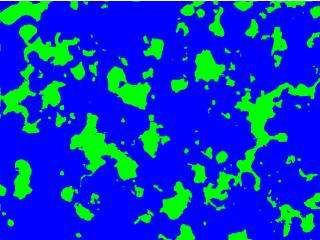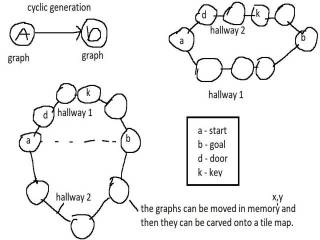-=+=- -=+=- -=+=- -=+=- -=+=- -=+=- -=+=- -=+=- -=+=- -=+=- -=+=- -=+=- -=+=- -=+=- -=+=- -=+=- -=+=- -=+=- -=+=- -=+=- -=+=- -=+=- -=+=- -=+=- -=+=- -=+=- -=+=- -=+=- -=+=- -=+=- (c) WidthPadding Industries 1987 0|697|0 -=+=- -=+=- -=+=- -=+=- -=+=- -=+=- -=+=- -=+=- -=+=- -=+=- -=+=- -=+=- -=+=- -=+=- -=+=- -=+=- -=+=- -=+=- -=+=- -=+=- -=+=- -=+=- -=+=- -=+=- -=+=- -=+=- -=+=- -=+=- -=+=- -=+=- SoCoder -> Snippet Home -> Generators Procedural, Random, Pseudo-Random.. Art, Levels, Creatures or Otherwise. If your code creates, then this is where it goes. Older --> Random Walker Mazelike maps(Godot script) Some Godot script for creating maps. Pakz , GDScript 3.2 Berzerk level generator Level generator from the classic game berzerk Pakz , Lua(codea) Ios Recursive map generator Pakz , Lua (codea) Ipados L Systems - plant L systems - string with turtle instructions Pakz , Lua - codea(ios)PHP Spritesheet Shuffler Jayenkai , Random Bag Get more predictable numbers Pakz , Monkey2 Rivers on a top down map. Create 2d Rivers Pakz , Monkey2Additive random line Texture Create a greyscale stone like texture Pakz , monkey2Maps or oilish paintings Smearing a additive brush Pakz , Monkey2Pixel Art Pine Tree(s) Generate sheets of pine trees(xmas?) Pakz , Monkey2 Seamless Grow Tiles(grass/desert etc) Create Tiles with zones method Pakz , Monkey2 Maps - Horizontal Line Puller Pull sections from a line to create maps Pakz , Monkey-X Map Generator Lines and Zones(grow) 2d Maps Pakz , Monkey-XHorizontal Hall with Rooms Generator Hall with Rooms generator Pakz , Monkey2 10 Print Chr (maze) Commodore 64 One line Maze Generator Pakz , Monkey-XZelda'ish Fantasy map generator Generate 2d fantasy maps Pakz , Monkey-XFlasks, Bottles Icons Create Bottle and/or flask like images Pakz , Monkey-XGem Sprites Jayenkai ,Cyclic Map Generator Generate maps where you travel/explore in a circle Pakz , Monkey-X Diffusion Limited Aggregation Maps Coding challenge from the coding train Pakz , Monkey-XSkylines Jayenkai Blitz MaxFlowers (Jan 2017) Make pretty flowers! Jayenkai Blitz Max Cross Platform Maze 2d - Recursive Backtracker Monkey 2 maze generator - tilemap Pakz Other Bush fire maps Create countries or provinces Pakz Other Grass like image generator Modified line function at random pos. Pakz Other Other Older -->